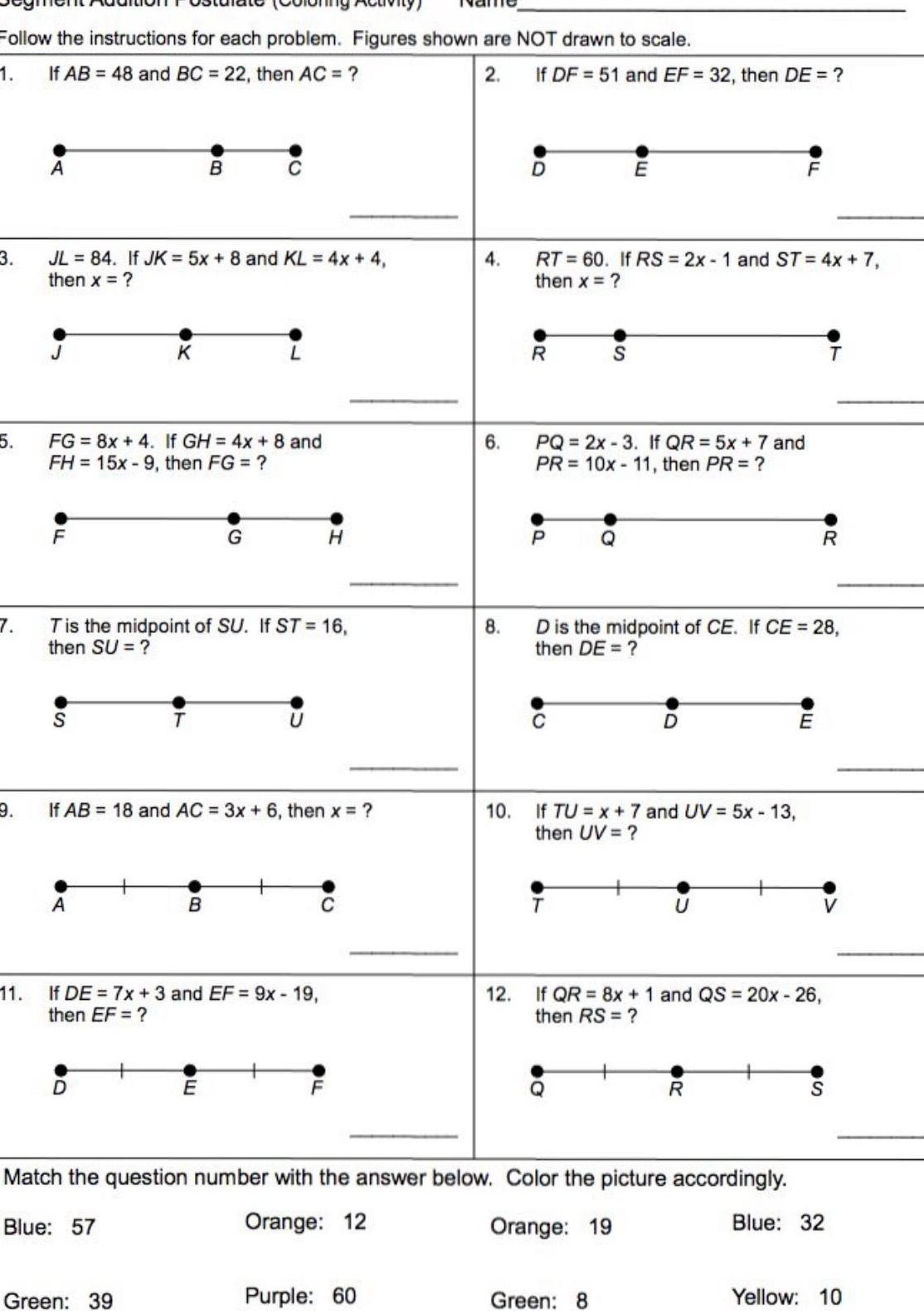Question:

# Follow the instructions for each problem Figures shown are

Last updated: 9/18/2023Follow the instructions for each problem Figures shown are NOT drawn to scale 1 If AB 48 and BC 22 then AC 2 3 5 7 9 11 A JL 84 If JK 5x 8 and KL 4x 4 then x FG 8x 4 If GH 4x 8 and FH 15x9 then FG F S B T is the midpoint of SU If ST 16 then SU A G If AB 18 and AC 3x 6 then x B Green 39 H If DE 7x 3 and EF 9x 19 then EF 4 Purple 60 6 8 If DF 51 and EF 32 then DE 12 D R RT 60 If RS 2x 1 and ST 4x 7 then x P PQ 2x 3 If QR 5x 7 and PR 10x 11 then PR E S 10 If TU x 7 and UV 5x 13 then UV T D is the midpoint of CE If CE 28 then DE Match the question number with the answer below Color the picture accordingly Blue 57 Orange 12 Orange 19 Green 8 F R If QR 8x 1 and QS 20x 26 then RS R E Blue 32 Yellow 10